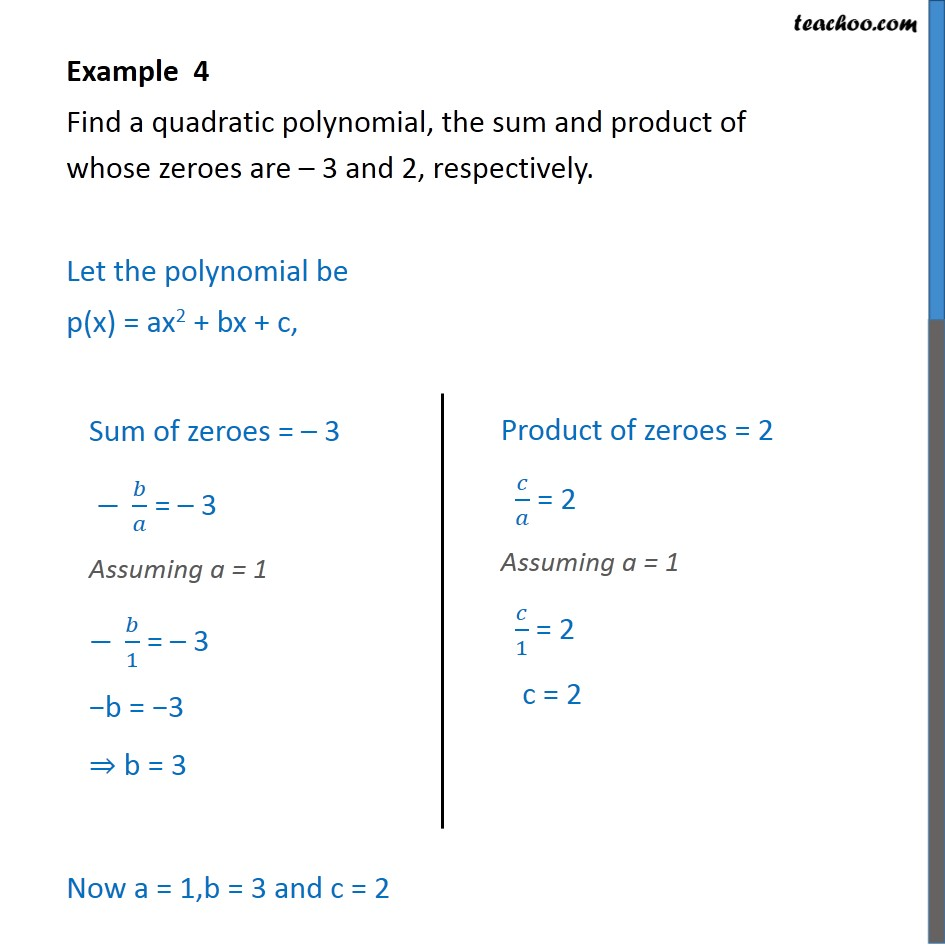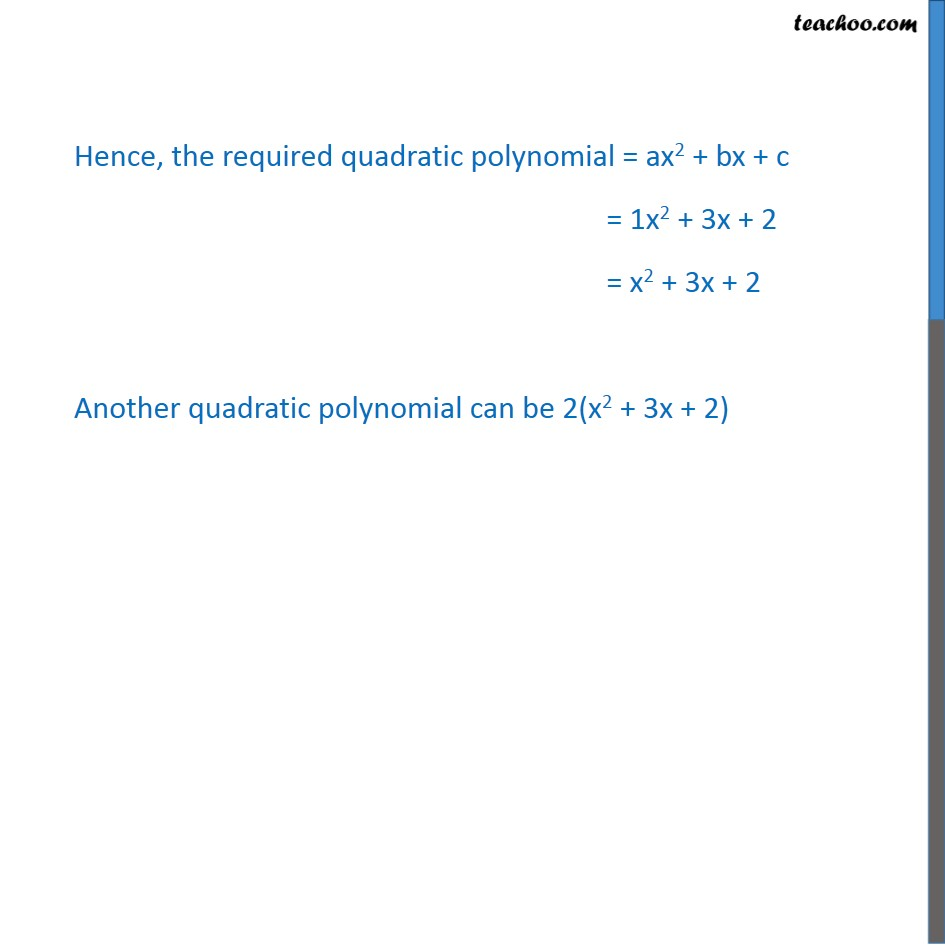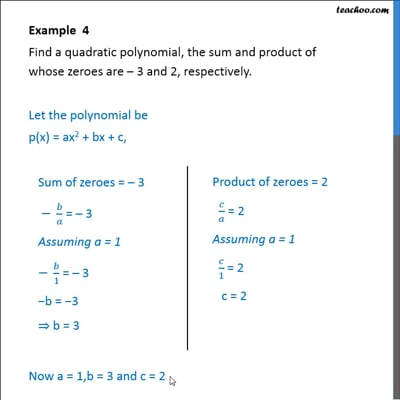Examples

Chapter 2 Class 10 Polynomials
Serial order wiseThis video is only available for Teachoo black users

Maths Crash Course - Live lectures + all videos + Real time Doubt solving!

### Transcript

Example 4 Find a quadratic polynomial, the sum and product of whose zeroes are – 3 and 2, respectively. Let the polynomial be p(x) = ax2 + bx + c, Sum of zeroes = -3 -b/a = -3 Assuming a = 1 -b/1 = -3 b = 3 Product of zeroes = 2 c/a = 2 Assuming a = 1 c/1 = 2 c = 2 Now a = 1,b = 3 and c = 2 Hence, the required quadratic polynomial = ax2 + bx + c = 1x2 + 3x + 2 = x2 + 3x + 2 Another quadratic polynomial can be 2(x2 + 3x + 2)